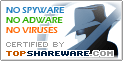# Digi]|[Trans 1.0

SNAPSHOT

Converter for different number systems

Windows AllPlatform :
\$0Price :
602 KBFile Size :
ScreenshotScreenshot :Popularity :Digi]|[Trans v1.0 is used to convert between diffrent number systems with a maximum base of 36.
One feature of Digi]|[Trans v1.0 is that you can also convert to number systems with rational bases. You can also convert from a latin number system.

Rational bases :

Rational bases are similar to integer bases. A carry happens if the numerator is reaching its maximum.

• StatAssist
View distribution graphs, calculate stat. moments, quantiles, and probabilities.
• Triple Integral Calculator Level 2
This calculator calculates triple integrals
• 3D-XplorMath-J
3D-XplorMath-J is a mathematical visualization application, i.e., a computer program for "seeing" and experimenting with a wide variety of mathematical objects or "exhibits."
• Math Solver II
An advance scientific calculator with a step-by-step solution system, and the ability to plot one or more expressions.
• Fret Specs
Fret Specs fret calculator for the stringed instrument craftsman and luthier
• BMP EMF Grapher
Graphics freeware with BMP, EMF, CSV support
• Quick Calculus
The simplest software for solving calculus.
• Crazy Math Game
Let your kids learn math Funny! Efficiently!
• Abilities Builder Add & Subtract Fractions